# PENGARUH PENGGUNAAN MULTIMEDIA DALAM PEMBELAJARAN INKUIRI TERHADAP HASIL BELAJAR IKATAN KIMIA DAN STRUKTUR MOLEKUL MAHASISWA PRODI PENDIDIKAN MATEMATIKA STKIP BUDIDAYA BINJAI

Seget, Tartiyoso (2011) PENGARUH PENGGUNAAN MULTIMEDIA DALAM PEMBELAJARAN INKUIRI TERHADAP HASIL BELAJAR IKATAN KIMIA DAN STRUKTUR MOLEKUL MAHASISWA PRODI PENDIDIKAN MATEMATIKA STKIP BUDIDAYA BINJAI. Masters thesis, UNIMED.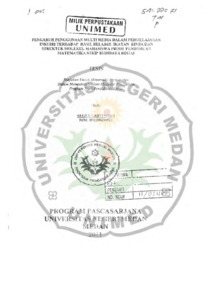Preview
Text
1-081188430013 Pengantar.pdf - Published Version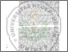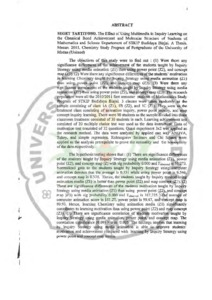Preview
Text
2-081188430013 Abstrak.pdf - Published Version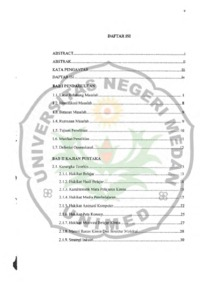Preview
Text
3-081188430013 Daftar isi.pdf - Published Version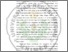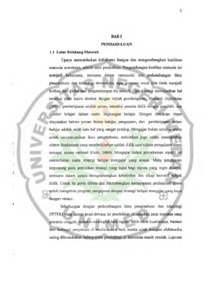Preview
Text
4-081188430013 BAb I.pdf - Published Version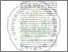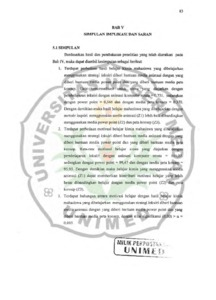Preview
Text
5-081188430013 BAb V.pdf - Published Version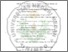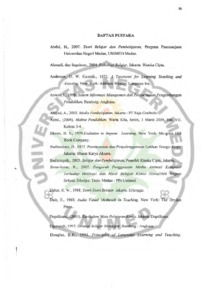Preview
Text
6-081188430013 Daftar Pustaka.pdf - Published Version

## Abstract

The objectives of this study were to find out : (1) Were there any significance differences of the achievement of the students taught by Inquiry Strategy using media animation (Z1) than using power point (Z2), and concept map (Z3). (2) Were there any significance differences of the students motivation in learning Chemistry taught by Inquiry Strategy using media animation (Z1) than using power point (Z2), and concept map (Z3). (3) Were there any significance correlations of the students taught by Inquiry Strategy using media animation (Z1) than using power point (Z2), and concept map (Z3). The research population were all the 2010/2011 first semester students of Mathematics Study Program of STKIP Budidaya Binjai. 3 classes were taken randomly as the sample consisting of class 1A (Z1), IB (Z2), and IC (Z3). They were as the treatment class consisting of animation inquiry, power point inquiry, and map concept inquiry learning, There were 90 students as the sample divided into three classroom treatment consisted of 30 students in each. Learning achievement test consisted of 20 multiple choice test was used as the data instrument. Data of motivation test consisted of 32 questions. Quasi experiment 3x2 was applied as the research method. The data were analyzed by applied one way ANOVA, Tukey, and simple regression. Kolmogorov Smirnov and Chi Square were applied as the analysis prerequisite to prove the normality and the homogenity of the data respectively.The hypothesis testing shows that : (1) There are significance differences of the students taught by Inquiry Strategy using media animation (Z1), power point (Z2), and concept map (Z) with sig probability 0.000 and Pobsaved is 55.273. Normalized gain to the students taught by Inquiry Strategy using computer animation denotes that the average is 0.751 while using power point is 0.546, and concept map is 0.370. Hence, the students taught by Inquiry method using animation media (Z1) is better than power point (Z2) and map concept (Z3). (2) There are significance differences of the students motivation taught by Inquiry Strategy using media animation (Z1) than using power point (Z2), and concept map )Z3) with sig probability 0.000 and Famed is 107.235.. The average ofcomputer animation score is 111.27, power point is 99.47, and concept map is99.93. Hence, learning Chemistry using animation media (Z1) significantlycontributes to learning motivation .than using power point (Z2) and map concept• (Z3). (3) There are significance correlation of students motivation taught byn Inquiry Strategy using media animation, power point, and concept map. Thecorrelation significance (0.00) > a is 0.005. The findings implies that learning by Inquiry Strategy using media animation is able to improve students motivation and achievement compared with learning by Inquiry Strategy using power point and concept map media.

Item Type: Thesis (Masters)
Contributors:
ContributionNameNIPView Item